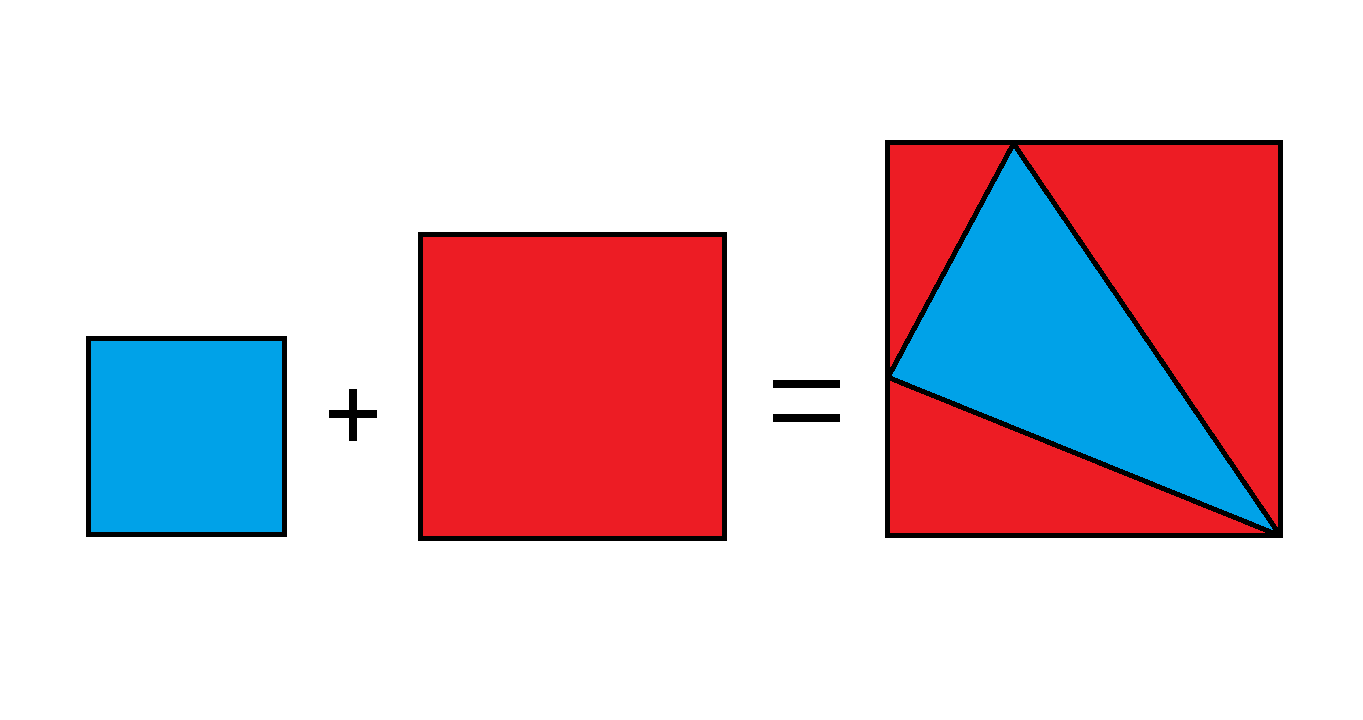# Pythagorean RiddleThe equation above shows that the sum of the areas of the blue and red squares on the left side is equal to the area of the big square on the right side. In addition, the area of the blue square equals the area of the blue triangle, and the area of the red square equals the combined area of the 3 red triangles. Each of the three squares has an integer side length.

If the 2 vertices of the blue triangle each divide the corresponding sides on which they lie into smaller integer lengths, what is the least possible difference between the red square's area and the blue square's area?

×